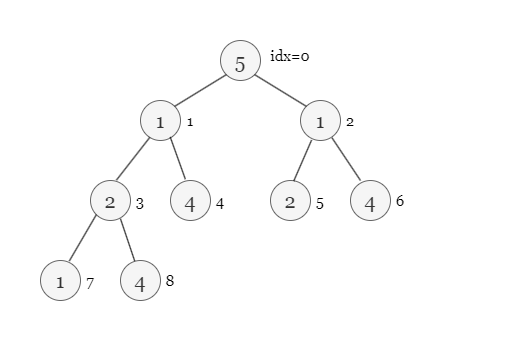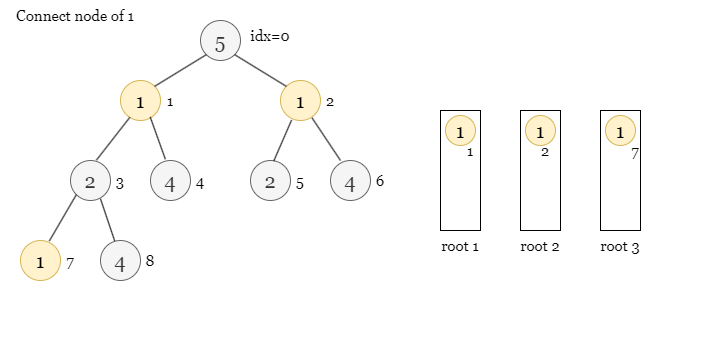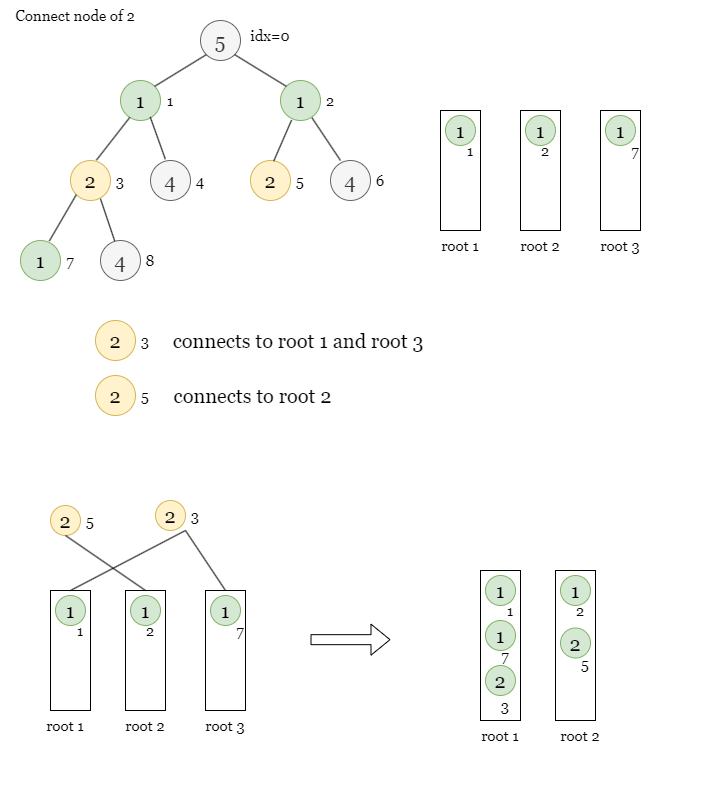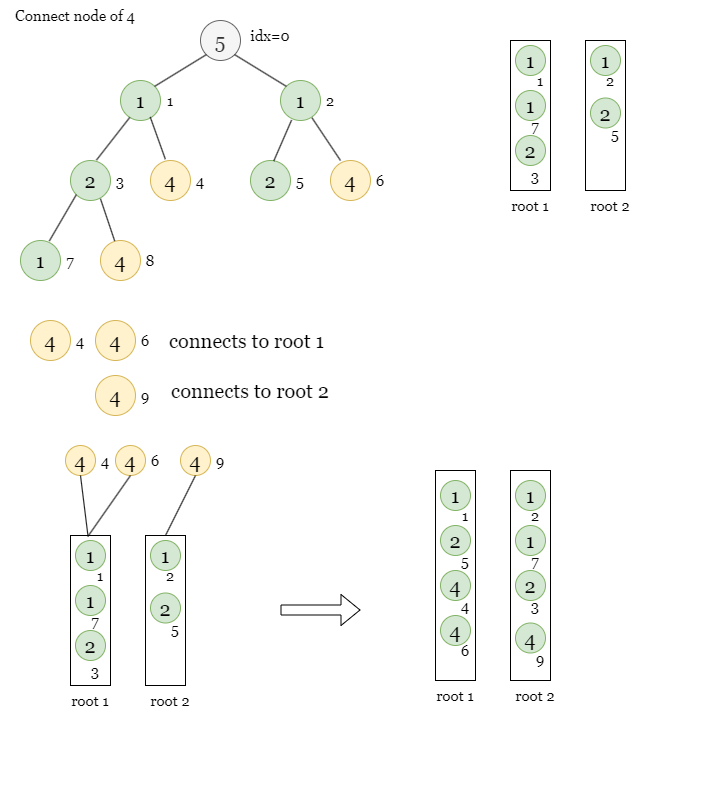Question: Google | September, 2022 | Recent Interview Questions | Special Paths | Count Pairs
0
Entering edit mode

Avg CTC: 12lpa for Google interns

Job Role: STEP intern

STEP Intern: Student Training Engineering Program

# Question 1

## Special Paths# Question 2

## Count Pairs4
Entering edit mode

# Solution to Problem 1

## Analysis

Since all the nodes in a path between the starting node and the terminating node have to be smaller than or equal to them, we can add the nodes in increasing order of their value. More formally, we will maintain a DSU and add the nodes in increasing order of their values. Now when we add a node (and its respective edges), we join two disjoint components (by the properties of a tree, every edge joins two disjoint components) and since all the nodes so far were smaller than or equal to current node in the value, the number of paths spanning through this node is equal to the product of frequency of the value of current node in the two components. Thus we can keep adding the nodes in the increasing order of their values and keep adding the number of new special paths formed.

Suppose we have a tree like below.Start with value = 1, we have 3 separate roots 1, 2 and 3.Now we move to values = 2, those two nodes connect to some neighbors with values = 1. Hence we get 1 special path from node 1 to 7 spanning through node 3Now we move to value = 4. We create one more special path from node 4 to node 8.## C++ Code

``````int numberOfSpecialPaths(vector&lt;int&gt;&amp; vals, vector&lt;vector&lt;int&gt;&gt;&amp; edges)
{
int n = vals.size();
map&lt;int,int&gt; freq[n];
for(int i=0; i&lt;n; i++) o[i] = i, par[i] = i, freq[i][vals[i]] = 1;
sort(begin(o),end(o),[&amp;](const int&amp; l, const int&amp; r){ return vals[l] &lt; vals[r]; });
for(auto&amp; edge:edges)
{
if(vals[edge] &gt; vals[edge]) swap(edge,edge);
}

int ans  = 0;
function&lt;int(int)&gt; find = [&amp;](int x)
{
return par[x] = (par[x] == x) ? x : find(par[x]);
};

auto merge = [&amp;](int a, int b)
{
int temp = vals[a];
a = find(a);
b = find(b);
if(a == b) return ;
ans += freq[a][temp] * freq[b][temp];
if(sizes[b] &gt; sizes[a]) swap(a,b);
sizes[a] += sizes[b];
par[b] = a;
if(freq[b].size() &gt; freq[a].size()) swap(freq[a],freq[b]);
for(auto&amp; [val,cnt]:freq[b]) freq[a][val] += cnt;
};
for(auto&amp; node:o)
{
}
return ans;
}
``````
3
Entering edit mode

# Solution to Problem 2

## Analysis

We need to count pairs `i,j (1 &lt;= i &lt; j &lt;= n)` satisfying the inequality `ai - aj + c &lt;= bi - bj + d`. We can rearrange the terms to obtain `ai - bi &lt;= aj - bj + d-c`. Thus for a given `j` we need to find number of `i` such that `i &lt; j` and `ai - bi &lt;= aj - bj + d-c`. We need to just query the number of elements smaller than some value discovered so far and keep adding the new elements. We can do this simply by iterating through the array elements and maintaining a Fenwick Tree (BIT)/Segment Tree/ C++ PBDS.

## PseudoCode

``````solve(a, b, n, c, d):
ans = 0
ds = new ordered_set&lt;int&gt;                                          //use either BIT/segment tree or PBDS
for j from 1 to n:
ans += ds.query_smaller_than_or_equal_to(a[j] - b[j] + d-c)    //as explained just query values smaller than a[j]-b[j]+d-c in the container so far
ds.insert(a[j] - b[j])                                         //and add the value corresponding to the jth element
return ans
``````
Entering edit mode
4

If you are not using PBDS, then I think you have to use Coordinate Compression in order to use values as indexes as Array elements can go upto `1e9`. At least this is how I did it. Also I think deciding which values to compress is something to think about here. I will explain a bit.

``````  (A[i] − A[j] + c) <= (B[i] − B[j] + d)
=> (A[i] - B[i]) + c <= (A[j] - B[j]) + d
=> A[i] + c <= A[j] + d         // A[i] := A[i] - B[i]
=> A[i] + (c - d) <= A[j];``````

So the values we are going to compress should be all the `A[i]` values and `A[i] + (c - d)` values, because these are the only values which will be compared against one another. So basically 2*N number of values. It's reasonable and works.

Since the official solution only has pseudo code, I will leave my solution with (Fenwick Tree + Coordinate Compression)

``````#include <bits/stdc++.h>
using namespace std;

template <class T>
struct CoordinateCompression {
public:
int n;
vector<T> helper, compressed;

CoordinateCompression(vector<T>& v) : helper(v), compressed(v) {
n = static_cast<int>(v.size());
sort(helper.begin(), helper.end());
helper.resize(unique(helper.begin(), helper.end()) - helper.begin());
for (int i = 0; i < n; ++i) {
compressed[i] = lower_bound(helper.begin(), helper.end(), compressed[i]) - helper.begin();
}
}

T& operator[](int index) {  // returns compressed value
assert(index >= 0 and index < n);
return compressed[index];
}

T& at(int index) {  // returns original value before compression
assert(index >= 0 and index < n);
return helper[compressed[index]];
}
};

template <typename T, class F = function<T(const T&, const T&)>>
struct FenwickTree {
public:
int n;
F merge;
vector<T> bit;

FenwickTree() {}

FenwickTree(int n, const F& f) : n(n), merge(f), bit(n, T(0)) {}

void init(vector<T>& array, const F& f) {
this->n = static_cast<int>(array.size());
this->merge = f;
bit.resize(n, T(0));
for (int i = 0; i < n; ++i) {
}
}

FenwickTree(vector<T>& array, const F& f) {
this->n = static_cast<int>(array.size());
this->merge = f;
bit.resize(n, T(0));
for (int i = 0; i < n; ++i) {
}
}

void add(int index, T delta) {
while (index < n) {
bit[index] = merge(bit[index], delta);
index = index | (index + 1);
}
}

T get(int index) {
T sum = 0;
while (index >= 0) {
sum = merge(sum, bit[index]);
index = (index & (index + 1)) - 1;
}
return sum;
}

T get(int l, int r) {
T ans = get(r);
if (l >= 1) ans -= get(l - 1);
return ans;
}
};

int main() {
ios::sync_with_stdio(false);
cin.tie(nullptr);
int t;
cin >> t;
while (t--) {
int n, c, d;
cin >> n >> c >> d;
vector<int> A(n);
for (auto& e : A) cin >> e;
for (int i = 0; i < n; ++i) {
int B;
cin >> B;
A[i] -= B;
}
long long ans = 0;
c = c - d;
vector<int> nums = A;
for (int i = 0; i < n; ++i) {
nums.push_back(A[i] + c);
}
unordered_map<int, int> mp;
for (int i = 0; i < ((int) nums.size()); ++i) {
mp[nums[i]] = i;
}
CoordinateCompression<int> ccp(nums);
int z = mp.size() + 2;
FenwickTree<int> ft(z, [&](int x, int y) { return x + y; });
for (int i = n - 1; i >= 0; --i) {
int num = A[i] + c;
int comp = ccp[mp[num]];
ans += ft.get(comp, z - 1);
int comp2 = ccp[mp[A[i]]];Similar Posts